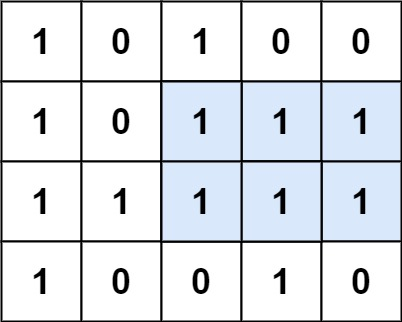304 North Cardinal St.
Dorchester Center, MA 02124

# Maximal Rectangle LeetCode Solution

## Problem – Maximal Rectangle LeetCode Solution

Given a `rows x cols` binary `matrix` filled with `0`‘s and `1`‘s, find the largest rectangle containing only `1`‘s and return its area.

Example 1:``````Input: matrix = [["1","0","1","0","0"],["1","0","1","1","1"],["1","1","1","1","1"],["1","0","0","1","0"]]
Output: 6
Explanation: The maximal rectangle is shown in the above picture.
``````

Example 2:

``````Input: matrix = [["0"]]
Output: 0
``````

Example 3:

``````Input: matrix = [["1"]]
Output: 1
``````

Constraints:

• `rows == matrix.length`
• `cols == matrix[i].length`
• `1 <= row, cols <= 200`
• `matrix[i][j]` is `'0'` or `'1'`.

## Maximal Rectangle LeetCode Solution in Python

``````def maximalRectangle(self, matrix):
if not matrix or not matrix:
return 0
n = len(matrix)
height =  * (n + 1)
ans = 0
for row in matrix:
for i in xrange(n):
height[i] = height[i] + 1 if row[i] == '1' else 0
stack = [-1]
for i in xrange(n + 1):
while height[i] < height[stack[-1]]:
h = height[stack.pop()]
w = i - 1 - stack[-1]
ans = max(ans, h * w)
stack.append(i)
return ans
``````

## Maximal Rectangle LeetCode Solution in Java

``````public class Solution {
public int maximalRectangle(char[][] matrix) {
if(matrix == null || matrix.length == 0 || matrix.length == 0) return 0;

int[] height = new int[matrix.length];
for(int i = 0; i < matrix.length; i ++){
if(matrix[i] == '1') height[i] = 1;
}
int result = largestInLine(height);
for(int i = 1; i < matrix.length; i ++){
resetHeight(matrix, height, i);
result = Math.max(result, largestInLine(height));
}

return result;
}

private void resetHeight(char[][] matrix, int[] height, int idx){
for(int i = 0; i < matrix.length; i ++){
if(matrix[idx][i] == '1') height[i] += 1;
else height[i] = 0;
}
}

public int largestInLine(int[] height) {
if(height == null || height.length == 0) return 0;
int len = height.length;
Stack<Integer> s = new Stack<Integer>();
int maxArea = 0;
for(int i = 0; i <= len; i++){
int h = (i == len ? 0 : height[i]);
if(s.isEmpty() || h >= height[s.peek()]){
s.push(i);
}else{
int tp = s.pop();
maxArea = Math.max(maxArea, height[tp] * (s.isEmpty() ? i : i - 1 - s.peek()));
i--;
}
}
return maxArea;
}
``````

## Maximal Rectangle LeetCode Solution in C++

``````class Solution {
public:
int maximalRectangle(vector<vector<char>>& M) {
if(!size(M)) return 0;
int ans = 0, m = size(M), n = size(M);
vector<vector<short>> dp(m+1, vector<short>(n+1));
for(int i = m-1; ~i; i--)
for(int j = n-1; ~j; j--)
dp[i][j] = M[i][j] == '1' ? dp[i][j+1] + 1 : 0;

for(int i = 0; i < m; i++)
for(int j = 0; j < n; j++)
for(int row = i, colLen = n; row < m && M[row][j] == '1'; row++)
ans = max(ans, (row-i+1) * (colLen = min(colLen, dp[row][j]*1)));

return ans;
}
};
``````
##### Maximal Rectangle LeetCode Solution Review:

In our experience, we suggest you solve this Maximal Rectangle LeetCode Solution and gain some new skills from Professionals completely free and we assure you will be worth it.

If you are stuck anywhere between any coding problem, just visit Queslers to get the Maximal Rectangle LeetCode Solution

Find on Leetcode

##### Conclusion:

I hope this Maximal Rectangle LeetCode Solution would be useful for you to learn something new from this problem. If it helped you then don’t forget to bookmark our site for more Coding Solutions.

This Problem is intended for audiences of all experiences who are interested in learning about Data Science in a business context; there are no prerequisites.

Keep Learning!

More Coding Solutions >>

LeetCode Solutions

Hacker Rank Solutions

CodeChef Solutions